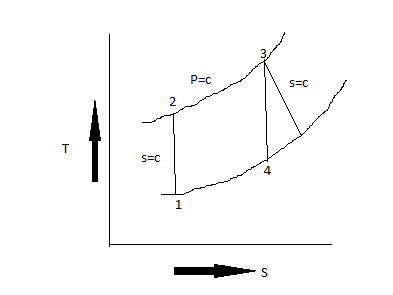# Consider a simple Brayton cycle that uses air as the working fluid to operate a gas-turbine power plant between the pressure limits of 100kPa and 900kPa. Air enters the compressor at 298K and leaves at 610K at a mass flow rate of 1200kg/min. The maximum cycle temperature is 1380K. The net power output from the cycle is measured experimentally to be 55 MW. Assuming constant properties (Cv,Cp, R, and k) for air at 300K:a) Sketch a T-s diagram for the cycleb) Determine the isentropic efficiency of the turbinec) Determine the thermal efficiency

Question
69 views

Consider a simple Brayton cycle that uses air as the working fluid to operate a gas-turbine power plant between the pressure limits of 100kPa and 900kPa. Air enters the compressor at 298K and leaves at 610K at a mass flow rate of 1200kg/min. The maximum cycle temperature is 1380K. The net power output from the cycle is measured experimentally to be 55 MW. Assuming constant properties (Cv,Cp, R, and k) for air at 300K:

• a) Sketch a T-s diagram for the cycle
• b) Determine the isentropic efficiency of the turbine
• c) Determine the thermal efficiency
check_circle

Step 1

Given information:

Step 2

(a)Draw the T-S diagram for the cycleStep 3

From the process 3-4 we ...

### Want to see the full answer?

See Solution

#### Want to see this answer and more?

Solutions are written by subject experts who are available 24/7. Questions are typically answered within 1 hour.*

See Solution
*Response times may vary by subject and question.
Tagged in

### Mechanical Engineering